Quantity: 0

Total: 0,00

0

# Cube

### Cube

This animation demonstrates the components (vertices, edges, diagonals and faces) of the cube, one of the Platonic solids.

Mathematics

Keywords

cube, vertex, edge, face, face diagonal, space diagonal, neighbouring faces, solid geometry, mathematics

Related items

### Scenes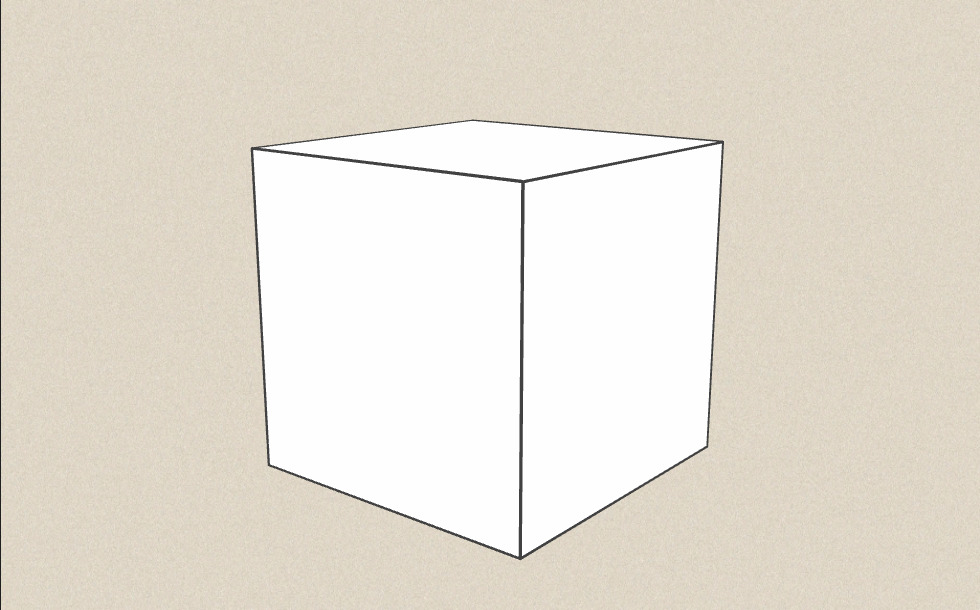### Cube

A cube is a rectangle that has edges of equal length. The cube is one of the five Platonic solids.

Properties

Number of vertices (V): 8

Number of edges (E): 12

Number of faces (F): 6

Euler´s formula is also valid for cubes:

F + V - E = 2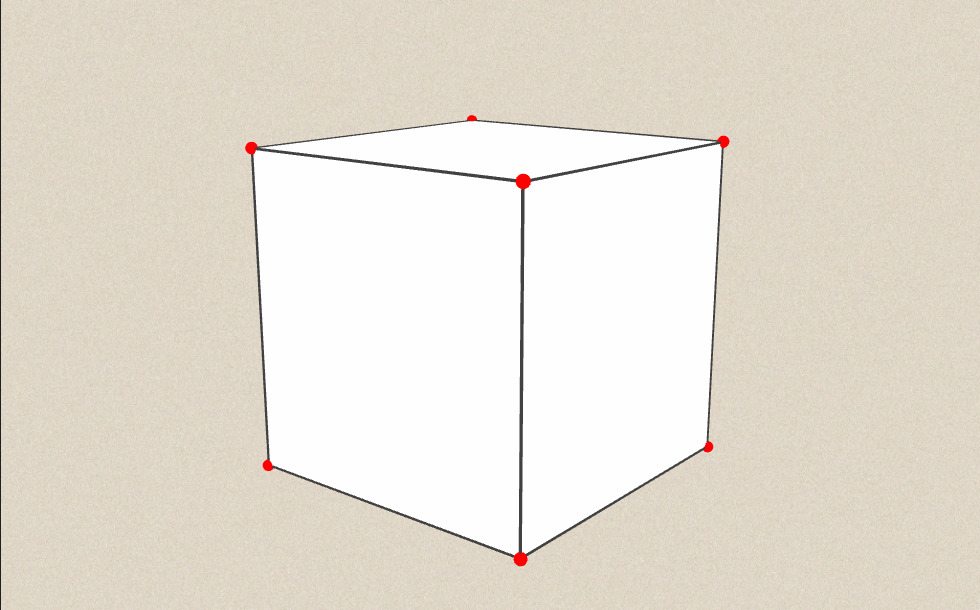• A
• B
• C
• D
• E
• F
• G
• H

## Vertices

Cubes have 8 vertices. These are marked by capital letters of the alphabet. To each vertex belong 3 edges and 3 faces.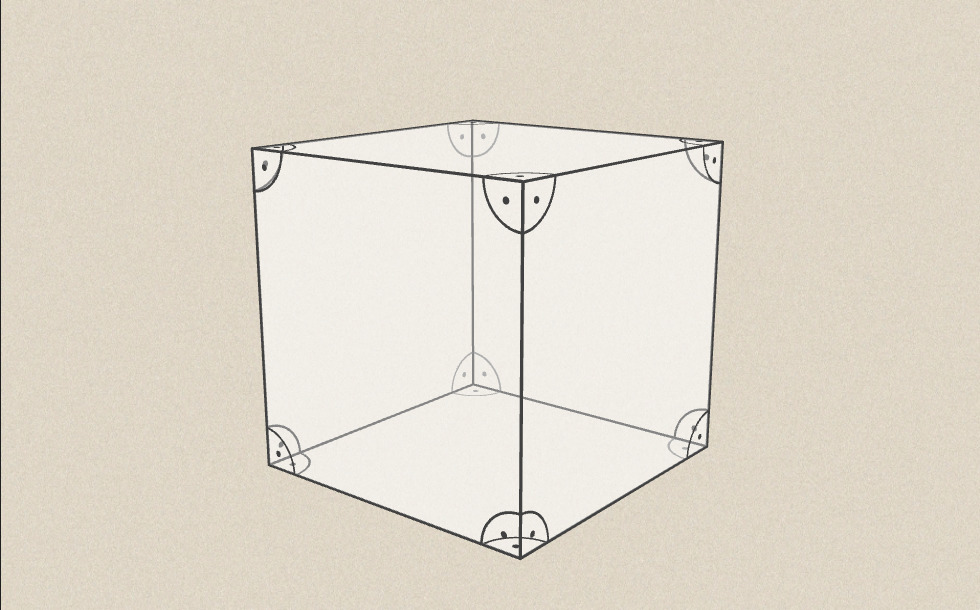• vertex
• edge
• face

## Edges

Cubes have 12 edges, each marked with two capital letters. Eg.: AB, DH,…

Two faces meet along one edge. Any two edges meeting at one vertex are perpendicular to each other. For each edge there are 3 edges that are parallel to it. All edges have 4 skew edges, but all of them are perpendicular to it.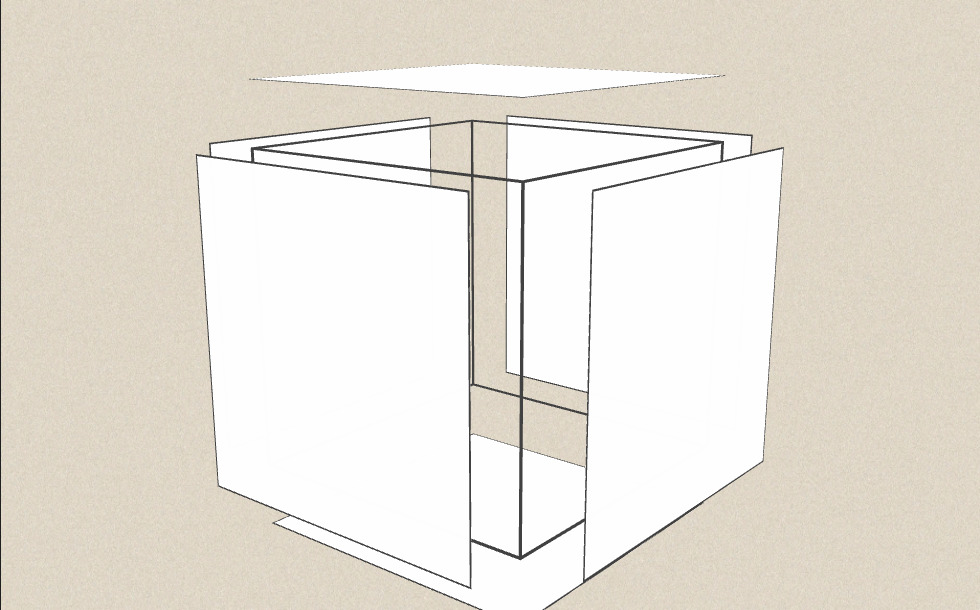## Faces

Cubes have 6 faces. All of them are square and any two of them are congruent. They are marked with the letters of their vertices e.g. ABCD, CDHG,…

Faces are pairwise perpendicular to each other.

Each face has exactly one face parallel to it, while all other faces are perpendicular to it.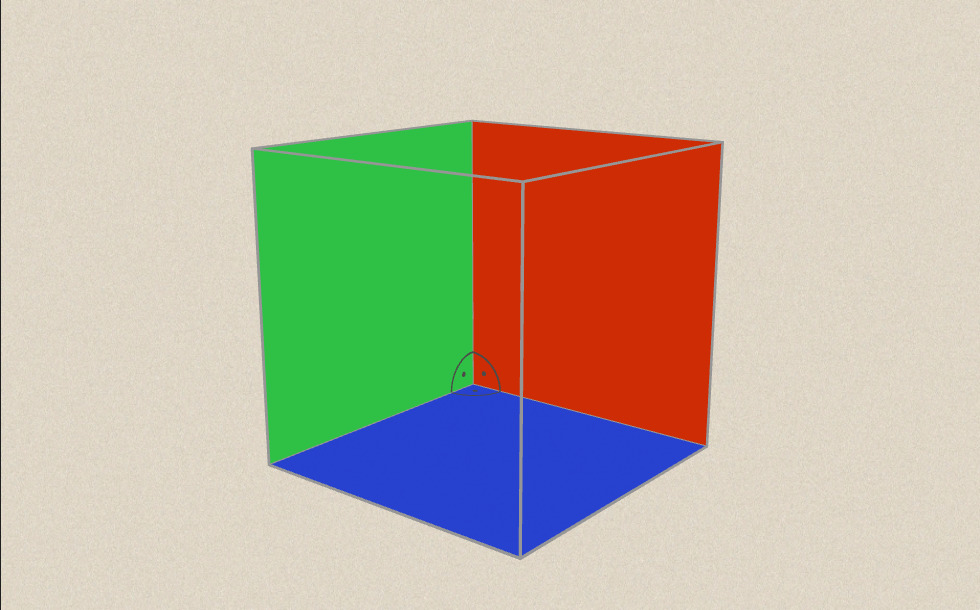### Neighbouring faces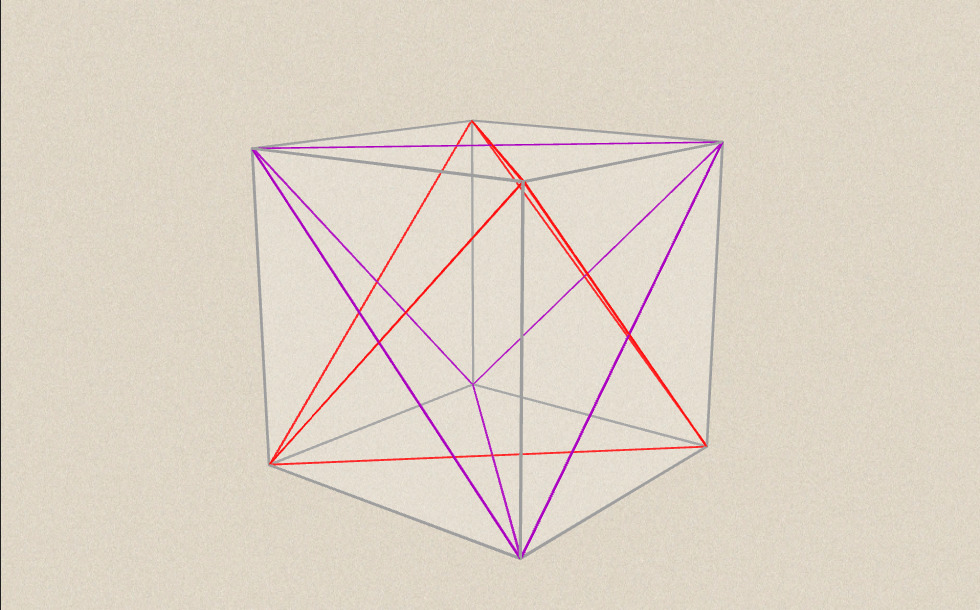## Face diagonals

Cubes have 6 faces and each face has 2 face diagonals. Consequently, cubes have 12 face diagonals of equal length.

Choosing 3 faces sharing one common vertex and drawing their face diagonals, which do not pass through the chosen vertex, results in a regular triangle.

Similarly, 6 face diagonals form a tetrahedron in the cube.

The remaining face diagonals form a tetrahedron congruent with the previous one.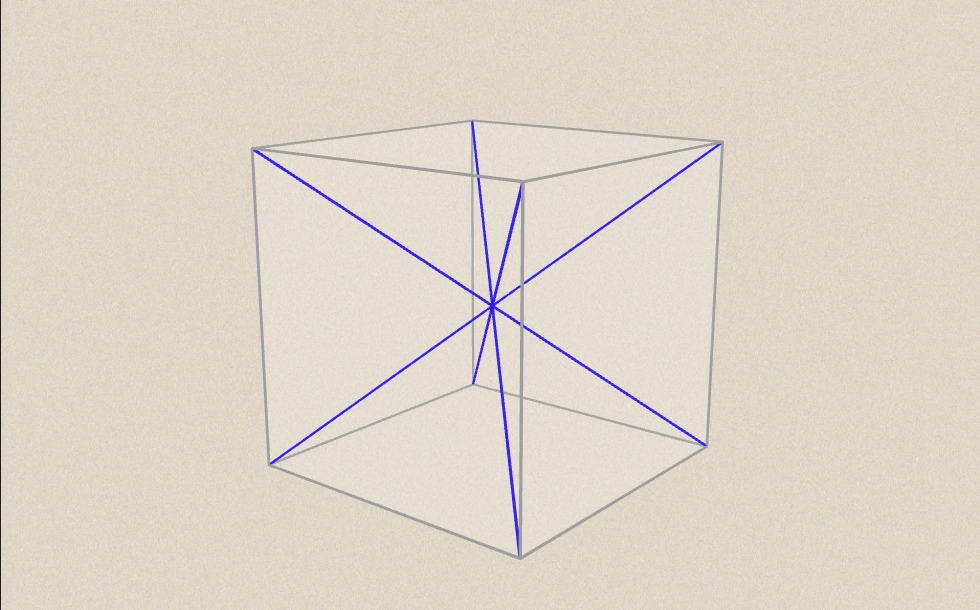## Space diagonals

Cubes have 4 space diagonals passing through one common point, i.e. the centre point of the cube. Space diagonals have the same length.

### Related items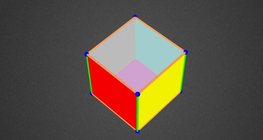#### Colouring a cube

Colouring the vertices, edges and faces of a given cube according to the criteria specified in the exercise.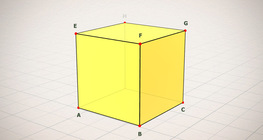#### Cube (exercises)

Edges, diagonals and faces of a cube can be identified by its vertices.#### Cube of cubes

An exercise about the regular hexahedron built from unit cubes to help deepen your knowledge of cubes.#### Net of a cube (exercises)

Not all nets consisting of 6 congruent squares are foldable into cubes.#### Building shapes (one colour)

Build 3D shapes from unit cubes with the help of several views.#### Cube sections (exercise)

Examining solids formed by the intersection of a cube and a plane.#### Cuboid

A cuboid is a polyhedron with six rectangular faces.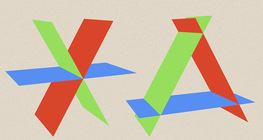#### Dividing Space into Regions by 3 Planes

Division of space by three intersecting planes in several different arrangements.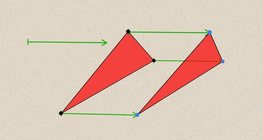#### Geometric transformations – translation

This animation demonstrates geometric translation in both plane and space.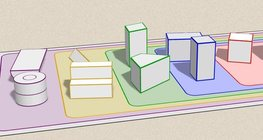#### Grouping of solids

This animation demonstrates various groups of solids through examples.#### Grouping of solids 1

This animation demonstrates various groups of solids through examples.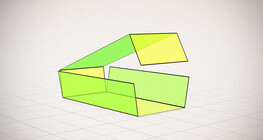#### Nets of a cuboid (exercises)

This animation demonstrates the different nets of a cuboid and includes a game.#### Perimeter, area, surface area and volume

This animation presents the formulas to calculate the perimeter and area of shapes as well as the surface area and volume of solids.#### Platonic solids

This animation demonstrates the five regular three-dimensional (or Platonic) solids, the best known of which is the cube.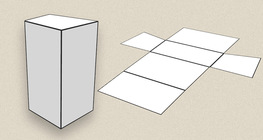#### Prisms

This animation demonstrates several types of prisms, from general to regular.#### Ratio of volumes of similar solids

This 3D scene explains the correlation between the ratio of similarity and the ratio of volume of geometric solids.#### Volume and surface area (exercise)

An exercise about the volume and surface area of solids generated from a ´base cube´.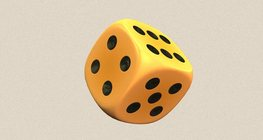#### Dice

Regular dice can be used for solving statistical and probability exercises.